﻿ 循序漸進介紹基於CommunityToolkit.Mvvm 和HandyControl的WPF應用端開發(3)--自定義用戶控制項_ZenDei技術網路在線

# 循序漸進介紹基於CommunityToolkit.Mvvm 和HandyControl的WPF應用端開發(3)--自定義用戶控制項### 1、自定義用戶控制項的應用場景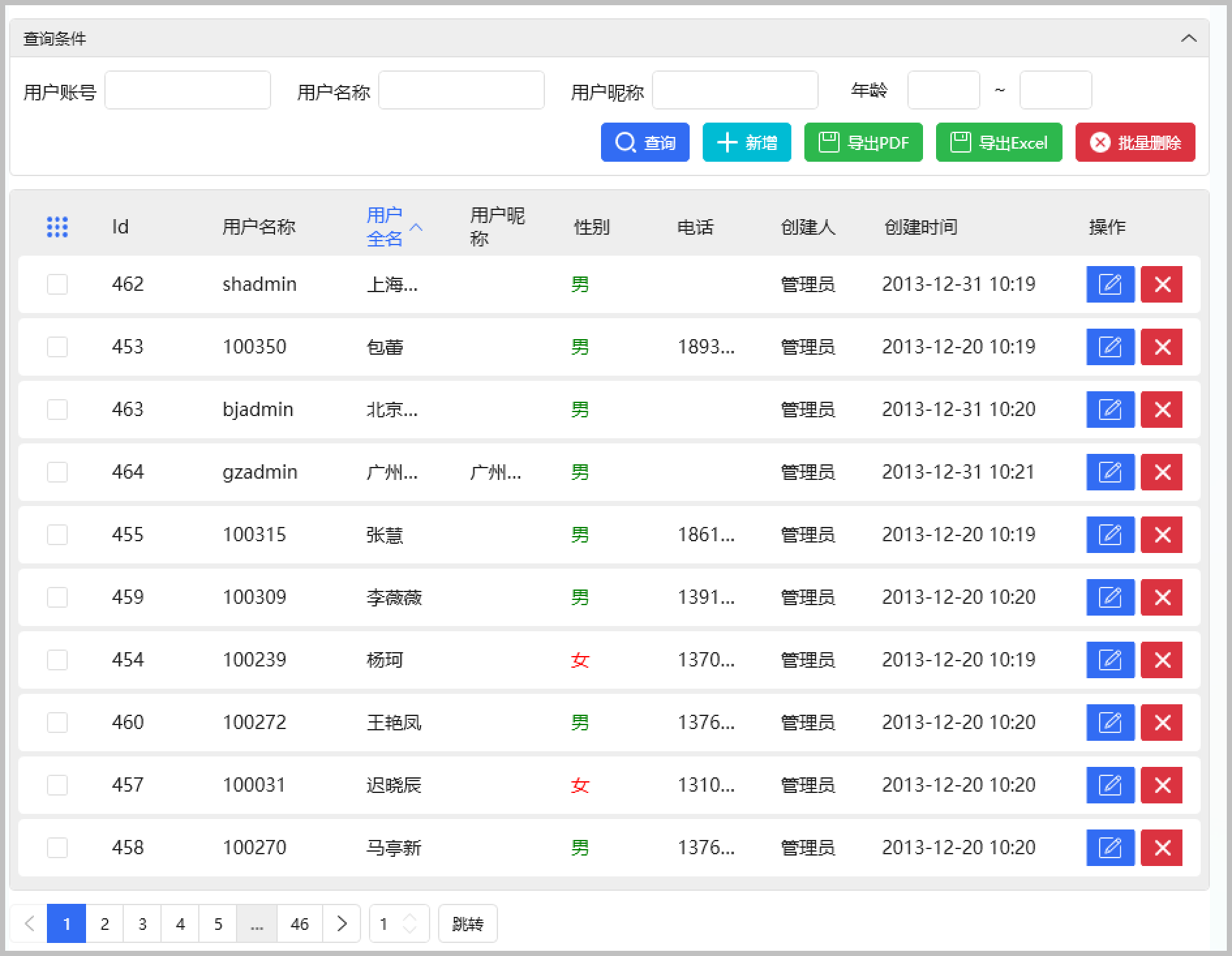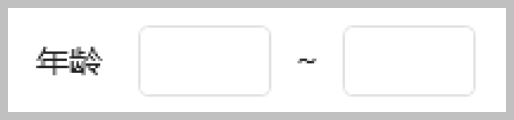### 2、自定義控制項的開發代碼

```<UserControl
x:Class="WHC.SugarProject.WpfUI.Controls.NumericRange"
xmlns="http://schemas.microsoft.com/winfx/2006/xaml/presentation"
xmlns:x="http://schemas.microsoft.com/winfx/2006/xaml"
xmlns:d="http://schemas.microsoft.com/expression/blend/2008"
xmlns:hc="https://handyorg.github.io/handycontrol"
xmlns:local="clr-namespace:WHC.SugarProject.WpfUI.Controls"
xmlns:mc="http://schemas.openxmlformats.org/markup-compatibility/2006"
Name="NumericRangeControl"
d:Background="Transparent"
d:DesignHeight="32"
d:DesignWidth="150"
d:Foreground="White"
mc:Ignorable="d">
<Grid MinWidth="150">
<Grid.ColumnDefinitions>
<ColumnDefinition Width="Auto" />
<ColumnDefinition Width="*" />
<ColumnDefinition Width="Auto" />
<ColumnDefinition Width="*" />
</Grid.ColumnDefinitions>
<TextBlock
Grid.Column="0"
Margin="10,0,10,0"
VerticalAlignment="Center"
Text="{Binding Path=Text, RelativeSource={RelativeSource AncestorType={x:Type local:NumericRange}}}" />
<TextBox
x:Name="txtStart"
Grid.Column="1"
Margin="5"
Text="{Binding Path=StartValue, Converter={StaticResource NumericConverter}, RelativeSource={RelativeSource AncestorType={x:Type local:NumericRange}}}" />
<TextBlock
Grid.Column="2"
Margin="5,0,5,0"
VerticalAlignment="Center"
Text="~" />
<TextBox
x:Name="txtEnd"
Grid.Column="3"
Margin="5"
Text="{Binding Path=EndValue, Converter={StaticResource NumericConverter}, RelativeSource={RelativeSource AncestorType={x:Type local:NumericRange}}}" />
</Grid>
</UserControl>```

` Text="{Binding Path=StartValue, Converter={StaticResource NumericConverter}, RelativeSource={RelativeSource AncestorType={x:Type local:NumericRange}}}" `

`Name="NumericRangeControl"`

```<TextBox
x:Name="txtStart"
Grid.Column="1"
Margin="5"
Text="{Binding Path=StartValue, Converter={StaticResource NumericConverter}, ElementName=NumericRangeControl}" />```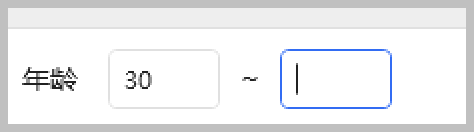如我們增加一個StartValue,那麼同時需要增加一個StartValueProperty的自定義依賴屬性。

```        /// <summary>
/// 開始值
/// </summary>
public decimal? StartValue
{
get { return (decimal?)GetValue(StartValueProperty); }
set { SetValue(StartValueProperty, value); }
}

public static readonly DependencyProperty StartValueProperty = DependencyProperty.Register(
nameof(StartValue), typeof(decimal?), typeof(NumericRange),

```        private static void OnStartValuePropertyChanged(DependencyObject d,DependencyPropertyChangedEventArgs e)
{
if (d is not NumericRange control)
return;

if (control != null)
{
var oldValue = (decimal?)e.OldValue;  // 舊的值
var newValue = (decimal?)e.NewValue; // 更新的新的值

var args = new RoutedPropertyChangedEventArgs<decimal?>(oldValue, newValue);
args.RoutedEvent = NumericRange.ValueChangedEvent;
control.RaiseEvent(args);
control.ValueChangedCommand?.Execute(null);
}
}```

```        /// <summary>
/// 數量改變命令
/// </summary>
public static readonly DependencyProperty ValueChangedCommandProperty =

/// <summary>
/// 數量改變命令
/// </summary>
public ICommand ValueChangedCommand
{
get { return (ICommand)GetValue(ValueChangedCommandProperty); }
set { SetValue(ValueChangedCommandProperty, value); }
}```

### 3、自定義控制項的使用

` xmlns:Controls="clr-namespace:WHC.SugarProject.WpfUI.Controls"`

``` <Controls:NumericRange
Text="年齡"
ValueChangedCommand="{Binding ViewModel.SearchCommand}" />```

```        <TextBox
x:Name="txtStart"
Grid.Column="1"
Margin="5"
KeyDown="txtStartEndValue_KeyDown"
Text="{Binding Path=StartValue, Converter={StaticResource NumericConverter}, ElementName=NumericRangeControl}" />

<TextBlock
Grid.Column="2"
Margin="5,0,5,0"
VerticalAlignment="Center"
Text="~" />

<TextBox
x:Name="txtEnd"
Grid.Column="3"
Margin="5"
KeyDown="txtStartEndValue_KeyDown"
Text="{Binding Path=EndValue, Converter={StaticResource NumericConverter}, RelativeSource={RelativeSource AncestorType={x:Type local:NumericRange}}}" />```

```        private void txtStartEndValue_KeyDown(object sender, KeyEventArgs e)
{
if(e.Key == Key.Enter)
{
var textBox = sender as System.Windows.Controls.TextBox;
if(textBox != null)
{
//切換焦點會觸發值更新命令
}
}
}```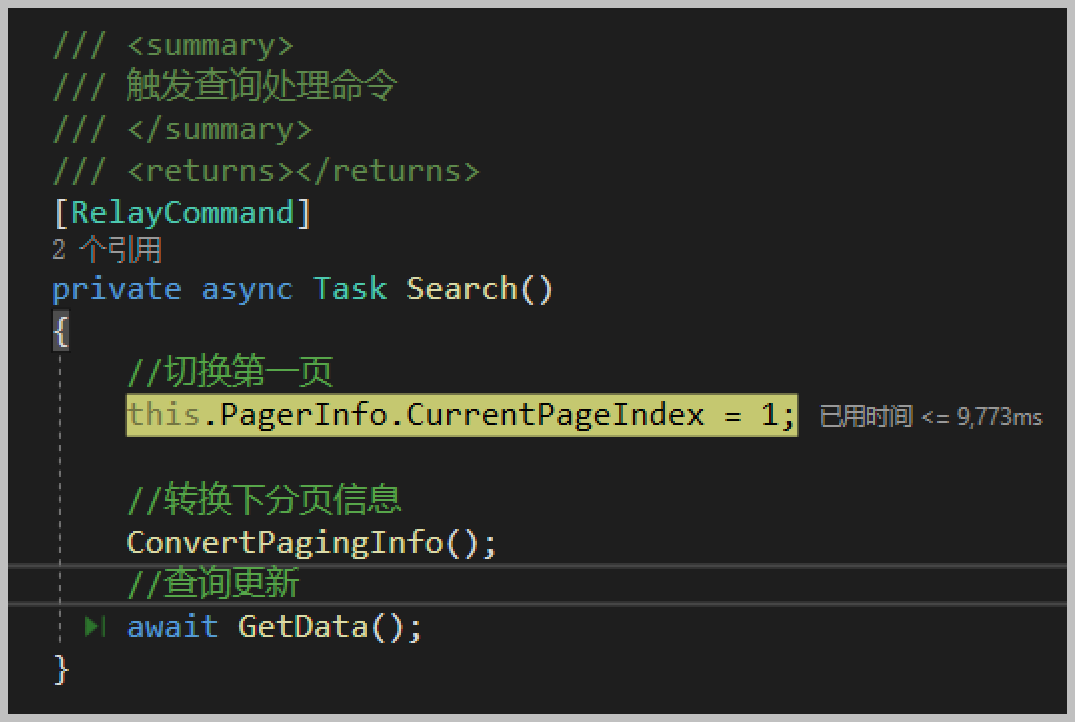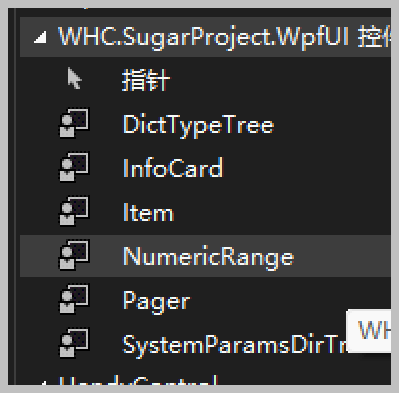至此，我們就實現了自定義控制項在頁面上的使用了，非常簡單。當然，我們也可以組合一些面板，來實現更加複雜的控制項呈現方式，可以設計一些圖表、文本內容的綜合展示，如下是其中的一個控制項的多層展示。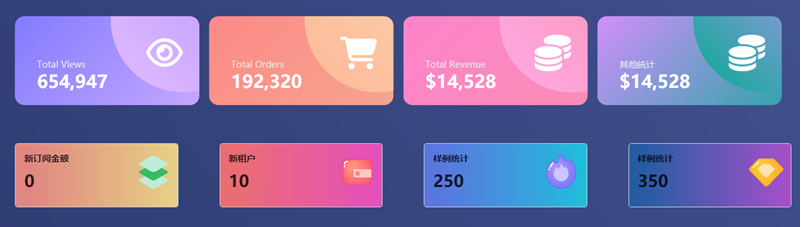專註於代碼生成工具、.Net/.NetCore 框架架構及軟體開發，以及各種Vue.js的前端技術應用。著有Winform開發框架/混合式開發框架、微信開發框架、Bootstrap開發框架、ABP開發框架、SqlSugar開發框架等框架產品。
轉載請註明出處：撰寫人：伍華聰  http://www.iqidi.com• Matplotlib 提供了大量配置參數，這些參數可以但不限於讓我們從整體上調整通過 Matplotlib 繪製的圖形樣式，這裡面的參數還有很多是功能性的，和其他工具結合時需要用的配置。 通過plt.rcParams，可以查看所有的配置信息： import matplotlib.pyplot as ...
• 大家好，我是TJ 今天給大家推薦一個開源協作式數字白板：tldraw。 tldraw的編輯器、用戶界面和其他底層庫都是開源的，你可以在它的開源倉庫中找到它們。它們也在NPM上分發，提供開發者使用。您可以使用tlDraw為您的產品創建一個臨時白板，或者將其作為構建自己應用的工具來使用。 線上體驗 tl ...
• 基於java學生考勤管理系統設計與實現，可適用於學生考勤系統，校園考勤，大學考勤管理，企業考勤系統，公司考勤系統，學校考勤系統。 ...
• 隨著JDK19的發佈，虛擬線程也逐漸被大家瞭解和使用，然而，主流java框架是否支持虛擬線程這一特性呢？咱們應用開發者如何通過框架使用虛擬線程特性？經過精心準備，欣宸原創在第一時間為您帶來詳細的實戰系列，與您共同學習 ...
• 大家好，又見面了。 在此前我的文章中，曾分2篇詳細探討了下JAVA中Stream流的相關操作，2篇文章收穫了累計 10w+閱讀、2k+點贊以及 5k+收藏的記錄。能夠得到眾多小伙伴的認可，是技術分享過程中最開心的事情。 吃透JAVA的Stream流操作，多年實踐總結 講透JAVA Stream的co ...
• 作者：美得讓人心動 來源：https://blog.csdn.net/gu131007416553/article/details/120934738 面試官在面試候選人時，如果發現候選人的簡歷中寫了在項目中使用了 MQ 技術（如 Kafka、RabbitMQ、RocketMQ），基本都會拋出一個問 ...
• 以下內容均來自Gitee的開源倉庫，具體的使用請移步Gitee：https://gitee.com/pojianbing/lazy-captcha 以下是我自己使用的具體方式 首先安裝NuGet包： Microsoft.Extensions.Caching.StackExchangeRedis La ...
• 首先需要安裝的NuGet包有： Microsoft.AspNetCore.Authentication.JwtBearer Swashbuckle.AspNetCore Swashbuckle.AspNetCore.Filters jose-jwt 大致是這些代碼放到項目中如果有報錯信息再去具體解決 ...